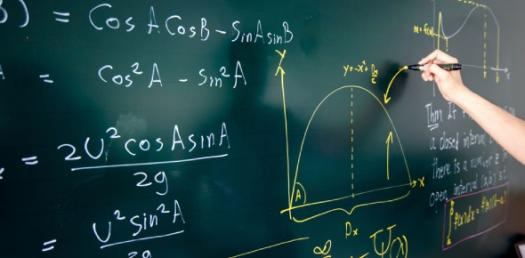# Ipm Maths Quiz G4

25 Questions | Total Attempts: 2157Settings.

• 1.
How many prime numbers are there between 1 and 100
• A.

25

• B.

50

• C.

37

• D.

None of the above

• 2.
Find the smallest 7 digit possible number using 6 , 2 , 9 , 0 , 8 , 7 , 4
• A.

9876420

• B.

0246789

• C.

2016789

• D.

None of the above

• 3.
Replace * in : 17+7 * 6 x 4
• A.

>

• B.

=

• C.

• D.

• 4.
Fill in the  * in the series 1 , 8 , 27 , *
• A.

36

• B.

64

• C.

54

• D.

I don't know

• 5.
Magic Square . find the value of  *
• A.

7

• B.

5

• C.

34

• D.

87

• 6.
XXVII + XI
• A.

XXVIII

• B.

XXXVI

• C.

XXXVIII

• D.

XVI

• 7.
• A.

21

• B.

25 square

• C.

15 square

• D.

None of the above

• 8.
798103 + 142047 + 2103
• A.

659873

• B.

456753

• C.

894123

• D.

942253

• 9.
Replace * with one digit 7*3   x   ____3* *99*    +    **3*0 26*81
• A.

9

• B.

3

• C.

7

• D.

1

• 10.
12 x 25 + 15 x 25 + 33 x 25 - 10 x 25
• A.

4942250

• B.

1250

• C.

1600456

• D.

1689710

• 11.
17a - 5 = 250 a = ?
• A.

246

• B.

16

• C.

160

• D.

15

• 12.
What is the sum of (2a + 5b) and (a + 7b)
• A.

6a + 12b

• B.

9a + 6b

• C.

3a + 12b

• D.

None of the above

• 13.
The number 4*3* is divisible by 11 both the *s are the same find *
• A.

2

• B.

5

• C.

9

• D.

8

• 14.
The G.C.D of two numbers is 15 and their L.C.M is 225. If one number is 45, what is the other one?
• A.

15

• B.

75

• C.

105

• D.

None of these

• 15.
Find out the proportional in which the denominator is 38
• A.

29/38

• B.

32/38

• C.

34/38

• D.

None of the above

• 16.
• A.

25/11

• B.

10/11

• C.

27/11

• D.

None of the above

• 17.
7.2 divided by 0.8
• A.

0.09

• B.

0.9

• C.

9

• D.

9.9

• 18.
10 kg rice costs rs 367 what is the price for 1 and a half kg of rice
• A.

67

• B.

55.05

• C.

55

• D.

36.7

• 19.
If ghandhi jayanti falls on sunday, on what day will children's day be?
• A.

THU

• B.

SUN

• C.

MON

• D.

TUE

• 20.
How many gross of books did a dealer buy if he bought 20160 books
• A.

165

• B.

789

• C.

140

• D.

49

• 21.
The sum of two angles is 180 degrees what are they called
• A.

Complimentary angles

• B.

Straight angles

• C.

Supplementary angles

• D.

Right angle

• 22.
Base = 0.8 dm height=20mm Area = ?
• A.

16 sq dm

• B.

16 sq cm

• C.

16 sq mm

• D.

1.6 sq cm

• 23.
How much of wire will be needed to fence a field 3 times over with dimensions 225m and 150 m
• A.

3 km

• B.

2.25 km

• C.

101250 m

• D.

1125 m

• 24.
What is the circumference of a circle with radius 2 cm take pi as 3.14
• A.

6.28 m

• B.

12.56 cm

• C.

12.49 cm

• D.

31.56 cm

• 25.
Find the area of a circle with radius 3 cm take pi as 3.14
• A.

31.46 sq cm

• B.

28.26 sq cm

• C.

18.84 sq cm

• D.

9.16 sq cm

Related TopicsBack to top